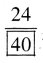# PSEB 4th Class Maths Solutions Chapter 3 Fractional Numbers Ex 3.2

Punjab State Board PSEB 4th Class Maths Book Solutions Chapter 3 Fractional Numbers Ex 3.2 Textbook Exercise Questions and Answers.

## PSEB Solutions for Class 4 Maths Chapter 3 Fractional Numbers Ex 3.2

1. Colour the given figures in such a way that they show equal fraction and also write their fraction in the given box :

Question 1.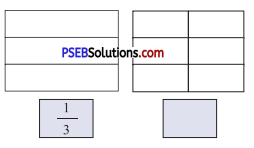Solution: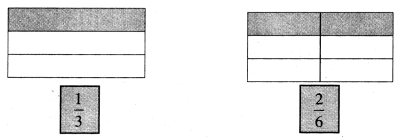Question 2.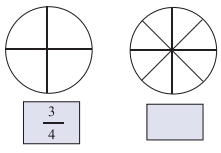Solution: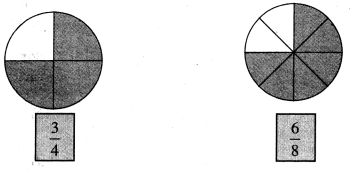2. Write next five equal fractions of the given each fraction :

Question 1.
$$\frac{1}{2}$$
Solution:
$$\frac{2}{4}$$, $$\frac{3}{6}$$, $$\frac{4}{8}$$, $$\frac{5}{10}$$, $$\frac{6}{12}$$

Question 2.
$$\frac{3}{4}$$
Solution:
$$\frac{6}{8}$$, $$\frac{9}{12}$$, $$\frac{12}{16}$$, $$\frac{15}{20}$$, $$\frac{18}{24}$$Question 3.
$$\frac{1}{3}$$
Solution:
$$\frac{2}{6}$$, $$\frac{3}{9}$$, $$\frac{4}{12}$$, $$\frac{5}{15}$$, $$\frac{6}{18}$$

Question 4.
$$\frac{2}{5}$$
Solution:
$$\frac{4}{10}$$, $$\frac{6}{15}$$, $$\frac{8}{20}$$, $$\frac{10}{25}$$, $$\frac{12}{30}$$

3. Fill in the blanks to make equal fractions to the given each fraction :

Question 1.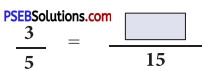Solution: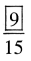Question 2.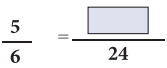Solution: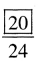Question 3.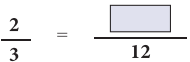Solution: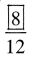Question 4.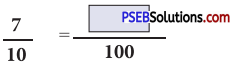Solution: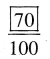Question 5.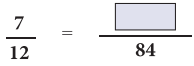Solution: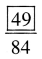Question 6.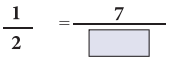Solution: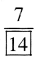Question 7.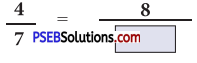Solution: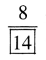Question 8.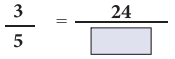Solution: